{ On Pythagorus Triples Expansion } Homepage

On a Quick Expansion Method for Pythagorus Triples

Summary and Key Findings :

Summary :

• In this paper , we shall develop 2 quick Expansion Formula for Pythagorus Triple :

• the Double-Up Expansion Formula :

• which involves only one ( 1 ) base { Pythagorus-Triple Triangle } ; and

• the One-On-One Expansion Formula :

• which involves two ( 2 ) base { Pythagorus-Triple Triangles } ,

• such as the { 3-4-5 Triangle } vs. the { 5-12-13 Triangle } .

Key Finding One :

• We first developed the { Double-Up Expansion Formula } applicable to any Pythagorus Triple .

For any Pythatgorus Triple { W / H / L } obeying :

•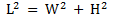we can always set up another Pythagorus Triple , with :

•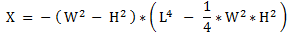•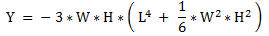•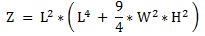so that :

•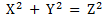Key Finding Two :

• We also developed the { One-On-One Expansion Formula } for combining 2 { Pythagorus Triples } :

We first bring in 2 { Pythagorus-Triple Triangles } resized to the same height ,

• and re-position the 2 triangles on the [ complex plane ] as per this set of 3 diagrams below :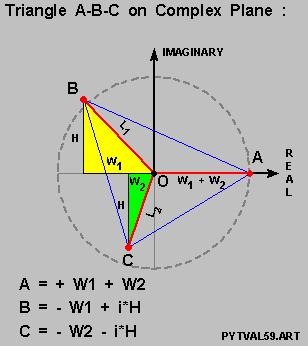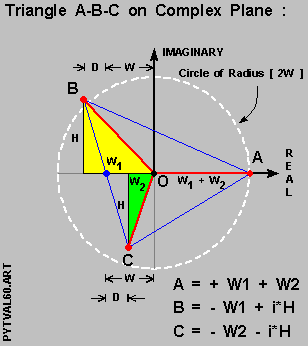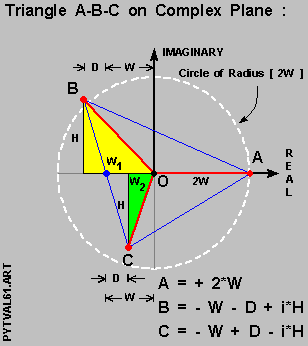so that :

• [ Point A ] is always positioned on the { Real Axis } and :

• positioned as such so that [ Point O ] the Point-Of-Origin is always the centroid of { Triangle A-B-C } .

We then set up the key values [ W ] / [ D ] / [ H ] via :

•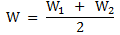•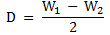•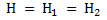so that :

• the assigned complex values of [ A ] / [ B ] / [ C ] are then :

•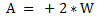•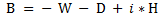•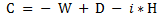And based on this , we then developed the { One-On-One Expansion Formula } for the Pythagorus Triples ,

• and the resultant { [ X ] / [ Y ] / [ Z ] Pythagorus Triple } is then :

•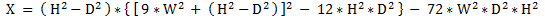•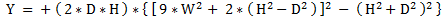•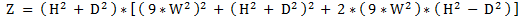• This looks rather complicated and we were able to revised this into this format below :

•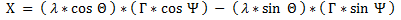•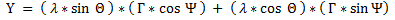•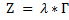at the end of Part VI of the paper .

Table of Content

Part I --- Introduction and Overview Part II --- Pythagorus Triples Basics Section I Introduction Section II An Overview of the Paper Section III Fermat's { Sum of 2 Squares } Revisited Section IV The Usual Procedure for Expanding Pythagprus Triples Section V Outline and Purpose for Party III Section VI Setting up the Random Triangle and its Reference Frame Section VII Creating the 3rd-Order Polynomial Equation Section VIII Solving the 3rd-Order Polynomial Equation Section IX Setting up the { Value-In-Question } - [ V-sub-IQ ] Section X Outline and Purpose for Party IV Section XI Results for the [ 3-4-5 / 5-12-13 ] Pythagorus-Triple Pair Section XII Results for the [ 3-4-5 / 8-15-17 ] Pythagorus-Triple Pair Section XIII Results for the [ 3-4-5 / 20-21-29 ] Pythagorus-Triple Pair Section XIV Results for the [ 3-4-5 / 12-35-37 ] Pythagorus-Triple Pair Section XV Results for the [ 5-12-13 / 8-15-17 ] Pythagorus-Triple Pair Section XVI Results for the [ 5-12-13 / 20-21-29 ] Pythagorus-Triple Pair Section XVII Results for the [ 5-12-13 / 12-15-37 ] Pythagorus-Triple Pair Section XVIII Results for the [ 8-15-17 / 20-21-29 ] Pythagorus-Triple Pair Section XIX Results for the [ 8-15-17 / 20-21-29 ] Pythagorus-Triple Pair Section XX Results for the [ 20-21-29 / 12-35-37 ] Pythagorus-Triple Pair Section XXI Summarizing on Part IV Section XXII Outline and Purpose for Part V Section XXIII Setting Up the new { Triangle A-B-C } for the Doubling-Up Process Section XXIV First Try at the Doubling-Up Process Section XXV Results of Doubling-Up Process thru to the Prime Number [ 233 ] Section XXVI Developing the Double-Up Expansion Formula ( I ) Section XXVII Developing the Double-Up Expansion Formula ( II ) Section XXVIII Developing the Double-Up Expansion Formula ( III ) Section XXIX Outline and Purpose for Part VI Section XXX Setting up the new { Triangle A-B-C } for One-On-One Expansion Section XXXI Developing the One-On-One Expansion Formula ( I ) Section XXXII Developing the One-On-One Expansion Formula ( II ) Section XXXIII Developing the One-On-One Expansion formula ( III ) Section XXXIV Developing the One-On-One Expansion formula ( IV ) Section XXXV Developing the One-On-One Expansion Formula ( V ) Section XXXVI Confirming the One-One-One Expansion Formula Section XXXVII Checking Out the One-On-One Expansion Formula Section XXXVIII Structure of the One-On-One Expansion Formula Section XXXIX Some Observations on Pythagorus Triples Section XXXX Concluding Remarks